# Maths Video Lesson 4 Revision eNotes# Contents

Lesson 4 – Quadratic Graphs 4

Free Maths Tutorial 4

Example 1 4

Example 1 Graph 5

Example 2 5

Example 3 7

Example 3 Graph 8

Example 4 9

Example 4 Graph 9

Method to sketch Quadratic graphs. 10

Example 5 11

Example 6 11

Intersecting Graphs 13

Example 7 13

Example 7 Graph 14

All revision notes have been produced by mockness ltd for iRevise.com.

Email: info@iRevise.com

All rights reserved; no part of this publication may be reproduced, stored in a retrieval system or transmitted, in any form or by any means, electronic, mechanical, photocopying, recording, reprinting, or otherwise without either the prior written permission of IRevise.com or a license permitting copying in the Republic of Ireland and United Kingdom issued by the copyright licensing Agency.

# Lesson 4 – Quadratic Graphs

## Free Maths Tutorial

These revision notes have been brought to you by an experienced Maths teacher - Mr Tom Nolan. These notes can be read in conjunction with the Free maths tutorial linked below. You will find this tutorial under iRevise.com, junior cert, maths, videos. These notes are suitable for Junior Certificate Students and covers quadratic graphs. There is a work sheet and solutions that are an add on to the notes and can also be purchased from iRevise.com.

## Example 1

Graph F(x) = 2x+3 From 3≤ x ≤ 3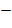Y = 2x+3

 X =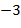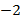0 1 2 3 2x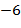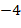0 2 4 6 +3 3 3 3 3 3 3 3 F(x) or Y=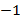1 3 5 7 9

Set Couples (,) (,1) () (0,3) (1,5) (2,7) (3,9)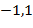## Example 1 Graph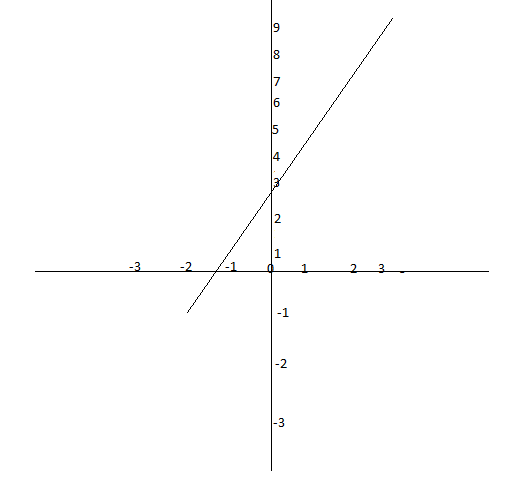## Example 2

Graph F(x) = x-4 from 0 ≤ x 4 ≤ 6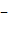x= 0 1 2 3 4 5 6 X 0 1 2 3 4 5 6 -4F(x)0 1 2

Couples (0-4) (1,-3) (2,-2) (3,-1) (4,0) (5,1) (6,2)

Example 2 Graph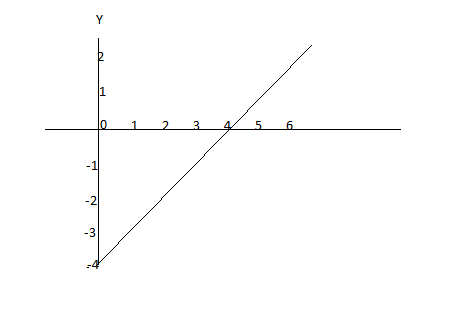Draw the same graph using X and Y intercepts.

Cuts the X-axis Y=0 Cuts Y-axis x=Y = X Y=X0=X Y=04 = X =(4,0) ...